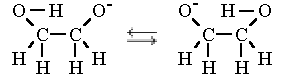# What exactly is barrier tunneling and potential barrier?

rozan977
How can we create a potential barrier? And how does it exactly acts as barrier in electron transfer?

Gold Member
By making a "step" in the potential energy. In the real world that usually means making a "sandwich" of 3 materials, the archtypical example being GaAs/AlGaAs/GaAs where the height of the barrier can be adjusted by varying the amount of aluminium in the middle layer.
This is how e.g. blue laser diodes are made.

Another option is to make a three terminal device where the middle terminal can be used as a gate; the height of the barrier is then set by adjusting the voltage applied to that gate.

rozan977
By making a "step" in the potential energy. In the real world that usually means making a "sandwich" of 3 materials, the archtypical example being GaAs/AlGaAs/GaAs where the height of the barrier can be adjusted by varying the amount of aluminium in the middle layer.
This is how e.g. blue laser diodes are made.

Another option is to make a three terminal device where the middle terminal can be used as a gate; the height of the barrier is then set by adjusting the voltage applied to that gate.

But how can we set the potential energy of barrier greater than the total energy in it? Also instead of reflection (as we may think in classical mechanics) how does a particle pass through the barrier (the amplitude of wave actually decreases exponentially,how is this possible)?
Answer to mine 2nd question too:
And how does it exactly acts as barrier in particle wave transfer?

Gold Member
I am not sure I understand the question.
Remember that potential energy is always something relative; where you set "zero" is arbitrary so there is nothing preventing us from making a barrier.

It is perhaps worth pointing out that in e.g. the GaAs/AlGaAs/GaAs structure I referred to above the difference in electron energy is just set by properties of the materials (there are tables which relate the amount of aluminium in percent to the energy in eV compared to pure GaAs). The "barrier" is a barrier simply because an electron would need more energy than is available in order to be able to jump up and populate an empty state in the valence band.

The answer to the second questions is: Because it can. Ultimately it is just how nature works simply because there is no such thing as a "classical particle". Everything in nature (including -at least in principle- you and me) have both "particle like" and "wavelike" properties and in structures where tunnelling can occur the latter dominates.

zenith8
Also instead of reflection (as we may think in classical mechanics) how does a particle pass through the barrier (the amplitude of wave actually decreases exponentially,how is this possible)?

It is impossible to explain the tunneling effect consistently using an interpretation of QM involving the wave function alone. All one can do is predict the probability of the tunneling occurring.

However, this is not so in the Bohm interpretation where there are particles in addition to the objectively existing wave function (with the wave exerting a force on the particles). This extra 'quantum force' can, under appropriate circumstances, boost a particle through the barrier. The force is proportional to the curvature of the amplitude of the wave field - and the rapid spacetime fluctuations of the field in the vicinity of the barrier sometimes mean that this force is very large.

The effective barrier encountered by the particle is thus not $$V$$ (the classical potential) but $$V+Q$$ (where $$Q$$ is the 'quantum potential'). This may be higher or lower than $$V$$ and may vary outside the 'true' barrier.

For more discussion and pretty pictures of trajectories of particle tunneling through barriers see p. 26-28 of lecture 3 of http://www.tcm.phy.cam.ac.uk/~mdt26/pilot_waves.html" [Broken].

Last edited by a moderator:
zenith8
The answer to the second questions is: Because it can.

Rozan977 - do you see how the Bohm interpretation is better?In the real world that usually means making a "sandwich" of 3 materials

Well, I guess in the 'usual' world of solid-state physics ;) The usual for a chemical physicist like me, is that the 'barrier' is the vacuum.

Say, I have an ethylene glycolate cation.. then it's plausible (I don't know if it's actually been measured in this specific case) that the hydroxyl hydrogen atom can 'flip-flop' between the two oxygens, like so:Now, to get from one oxygen to the other, the hydrogen atom has to pass through the vacuum - weakening its bond before forming a new one. So it goes up in energy and then down to where it was. (since the two structures are equivalent). If you displayed energy in terms of the 1-dimensional coordinate of the hydrogen atom position it'd be two valleys with a hump in-between: The potential barrier. (which'd be something like 0.1 eV in this case, relative the 'valleys' at each oxygen)

But since hydrogen is a very small atom, it can tunnel quantum-mechanically! In other words, it goes through the barrier: from one oxygen to the other without passing any points in-between! This effect is actually big enough to be measurable when it comes to some hydrogen-transfer reactions (they occur faster than if the hydrogen only went 'over' the barrier). Although this specific example is my own invention.

I believe hydrogen and deuterium are the heaviest things tunneling has been observed for, since it's strongly dependent on mass. In electon transfers it's so significant it always has to be taken into account.